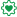# New to Qlik Sense

Discussion board where members can get started with Qlik Sense.

Announcements
BI & Data Trends 2021. Discover the top 10 trends emerging in today. Join us on Dec. 8th REGISTER
cancel
Showing results for
Did you mean:
HighlightedEmployee

## Standard Deviation

I need to calculate the average time between 2 dates and then calculate the average minus 1 standard deviation and then the average plus 1 standard deviation.

This is the expression I am using to calculate the average.

This seems to work ok.

Not sure how to calculate the STD and do the above?

Thanks

1 Solution

Accepted Solutions
HighlightedSpecialist II

Try the same formula replacing AVG with STDEV for the standard deviation.  Add/Subtract from the AVG value.